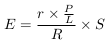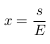# Regrexit Map Detail

How were the levels of regrexit on the map calculated? Read on to find out.

## Data

We combined the number of remain votes cast in each Local Authority District (LAD) with the number of names added to the petition in each Parliamentary Constituency (PCON) calling for a second EU Referendum.

The referendum results were extracted from the Guardian website. The petition resuls were taken from the government's official petition website.

PCON boundaries were provided by Martin Chorley's GeoJSON Data. Conversion between LAD and PCON regions was provided by the UK Data Service Census Support.

## Calculation

To establish the level x of regrexit we first calculate E, the expected number of names on the petition in a PCON. This is determined using the following formula.where r is the number of remain votes in the containing LAD, R is the total number of remain votes cast in the referendum, S is the total number of names added to the petition, P is the area of the PCON and L is the area of its containing LAD.

Having calculated this we can then calculated the level of regrexit as the proportion between the expected and actual number of names on the petition as follows.where s is the actual number of names on the petition for the particular PCON.

The resulting value allows us to measure whether the electorate has changed its mind or not since the referendum result. A value x < 1.0 suggests that there was a consolidation of the result for that region. A value x > 1.0 suggests a level of regret over the result. A value of x ≈ 1.0 suggests that the number of names on the petition is in line with the remain vote in the referendum.

## Assumptions

In practice we're making a lot of assumptions in this calculation. We assume that across the country the level of regret broadly cancels out; in other words, we are really only measuring the relative level of regret across the regions. We also have to assume that adding a name to the petition suggests dissatisfaction with the result in a particular constituency (rather than the final result as a whole).

The assumption that the ratio between the names on the petition and remain votes is a measure of satisfaction/dissatisfaction with the result is also questionable. For example, it could simply be that there are disparities between the disposition to sign a petition in one region compared to another.

The data on referendum votes is provided per LAD, whereas the petition data is provided per PCON. We therefore have to approximate the number of referendum votes by distributing them in proportion to the relative size of each PCON within the larger LAD. We use the relative number of postcodes in each region for this, which isn't an ideal measure, but should provide a reasonable approximation.

It's also worth pointing out that our analysis ignores the number of leave votes entirely. This is an unfortunate consequence of the fact we only have data on how many people want a rerun of the referendum (there's no petition calling for parliament not to have a second referendum).

In short, we have to make a lot of assumptions. By the time we reach a final number, the application of a decent quantity of salt is advisable.

## Where are the values for Northern Ireland?

For some reason the Guardian data didn't contain the details for Northern Ireland or other territories outside Great Britain such as Gibralta, so we weren't able to generate results for these areas.